# Adding normal and reverse faults to an impedance model with Python

This is a short and sweet follow-up to yesterday’s post Making many synthetic seismic models in Python, in which I want to show how to add oblique faults to an impedance model, as opposed to a vertical one.

In the pseudo-code below, with the first line of code I select one of the impedance models from the many I created, then in lines 2 and 3, respectively, make two new versions, one shifted up 5 samples, one shifted down 5 samples. The impedance values for the extra volume added – at the bottom in the first case, the top in the second – are derived from the unshifted impedance model.

Next, I make two copies of the original, call them normal and reverse, and then replace the upper triangle with the upper triangle of the shifted down and shifted up versions, respectively.

```unshifted = impedances[n]
up = sp.ndimage.interpolation.shift(unshifted, (5,0), cval=unshifted[0,0] * 0.9)
down = sp.ndimage.interpolation.shift(unshifted, (-5,0), cval=unshifted[0,-1] * 0.8)
normal = copy.deepcopy(unshifted)
reverse = copy.deepcopy(unshifted)
sz = len(unshifted)-1
normal[np.triu_indices(sz)] = up[np.triu_indices(sz)]
reverse[np.triu_indices(sz)] = down[np.triu_indices(sz)]```

Done!
The results are shown in the figure below.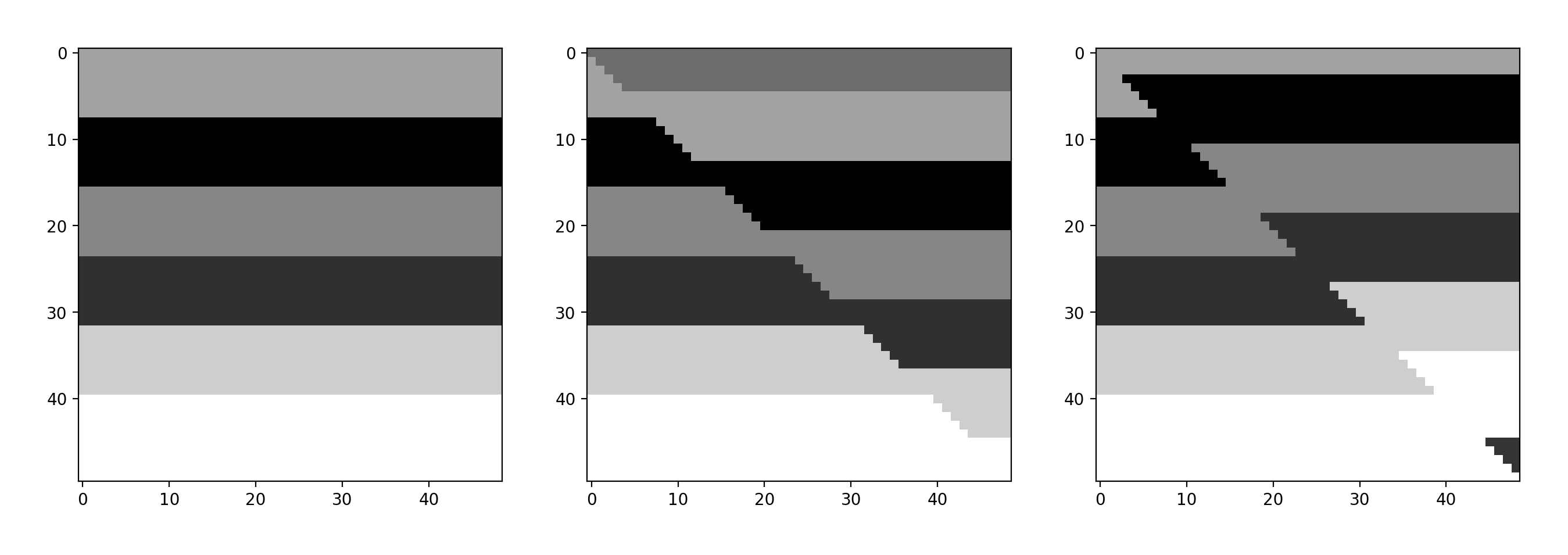Left: unfaulted impedance model; center, model with normal fault; right, model with reverse fault.

# Making many synthetic seismic models in Python

In this short post I show how to adapt Agile Scientific‘s Python tutorial x lines of code, Wedge model and adapt it to make 100 synthetic models in one shot: X  impedance models times X wavelets times X random noise fields (with I vertical fault).

N.B. the code is optimized for Python 2.7.

I begin by making a 6-layer model, shown in Figure 1:

```model = numpy.zeros((50,49), dtype=np.int)
model[8:16,:] = 1
model[16:24,:] = 2
model[24:32,:] = 3
model[32:40,:] = 4
model[40:,:] = 5```Figure 1. Initial 6-layer model

next I make some Vp-rho pairs (rock 0, rock 1, … , rock5):

```rocks = numpy.array([[2700, 2750],  # Vp, rho
[2400, 2450],
[2600, 2650],
[2400, 2450],
[2800, 3000],
[3100, 3200],])```

and then create 10 slightly different variations of the Vp-rho pairs one of which are is shown in Figure 2:

```rnd = numpy.random.rand(10,6,2)*0.2
manyrocks = np.array([rocks + rocks*rn for rn in rnd], dtype=np.int)
earth = manyrocks[model]```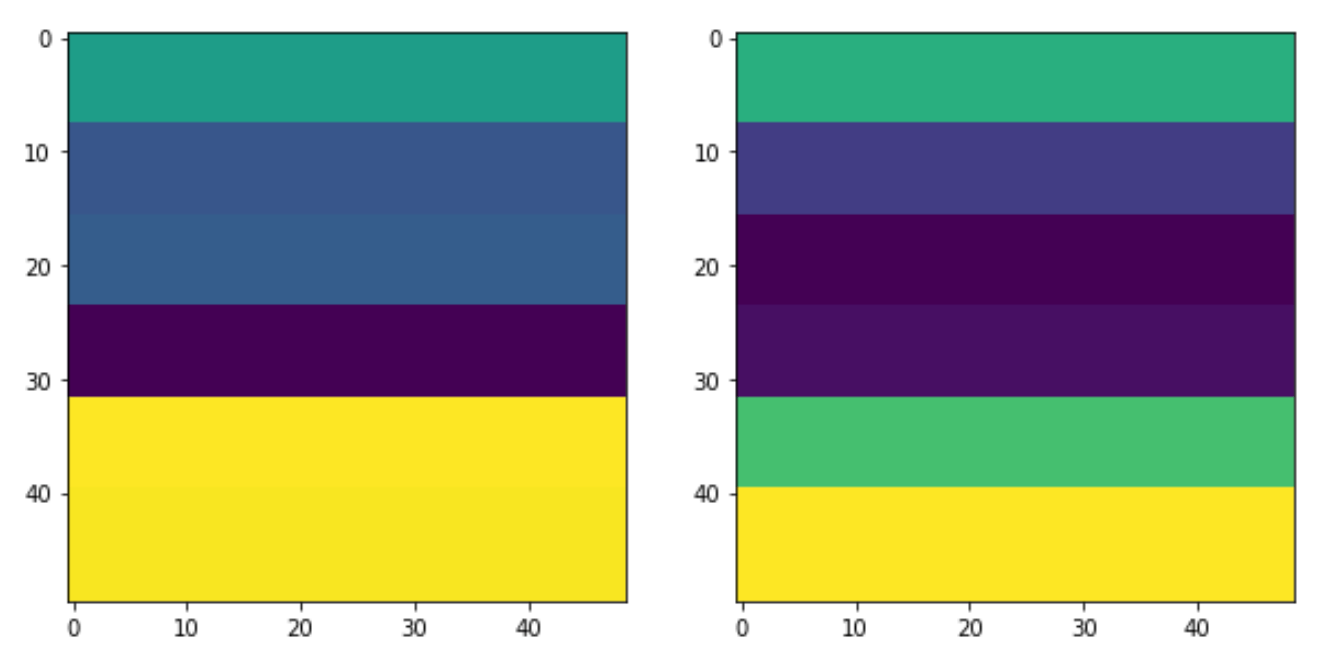Figure 2. A Vp-rho pair (earth model)

at which point I can combine Vp-rho pairs to make 10 impedance models, then insert a vertical fault with:

```impedances = [np.apply_along_axis(np.product, -1, e).astype(float) for e in earth]# Python 2
faulted = copy.deepcopy(impedances)
for r, i in zip(faulted, np.arange(len(faulted))):
temp = np.array(r)
rolled = np.roll(np.array(r[:,:24]), 4, axis = 0)
temp[:,:24]=rolled
faulted[i]=temp```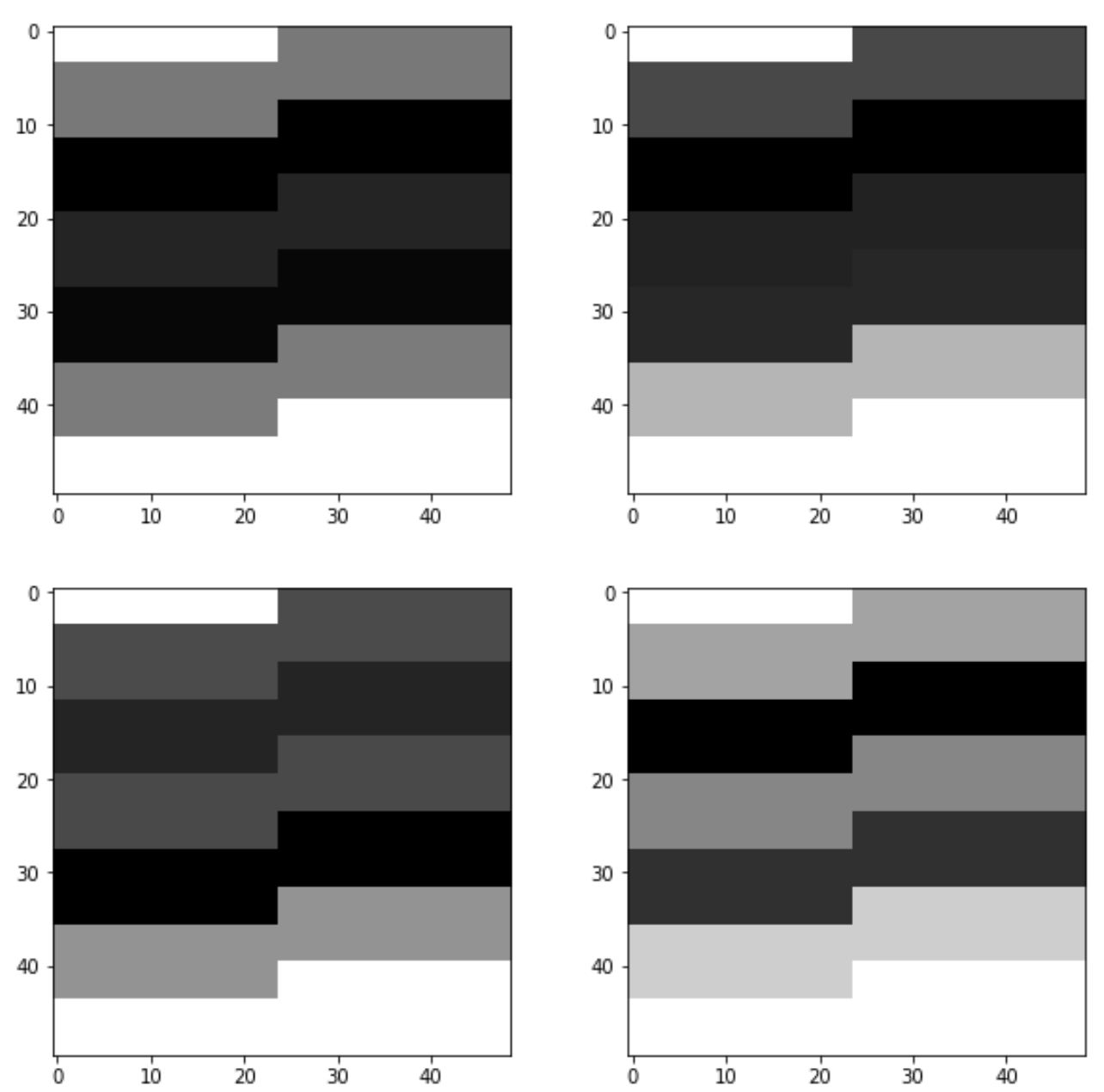Figure 3. Four faulted impedance models.

next I calculate reflection coefficients (Figure 4)and convolve them with a list of 10 Ricker wavelets (generated using Agile’s Bruges) to make synthetic seismic models, shown in Figure 5.

```rc =  [(flt[1:,:] - flt[:-1,:]) / (flt[1:,:] + flt[:-1,:]) for flt in faulted]
ws = [bruges.filters.ricker(duration=0.098, dt=0.002, f=fr)
for fr in [35, 40, 45, 50, 55, 60, 65, 70, 75, 80]]
synth = np.array([np.apply_along_axis(lambda t: np.convolve(t, w, mode='same'), axis=0,
arr=r) for r in rc for w in ws ])
```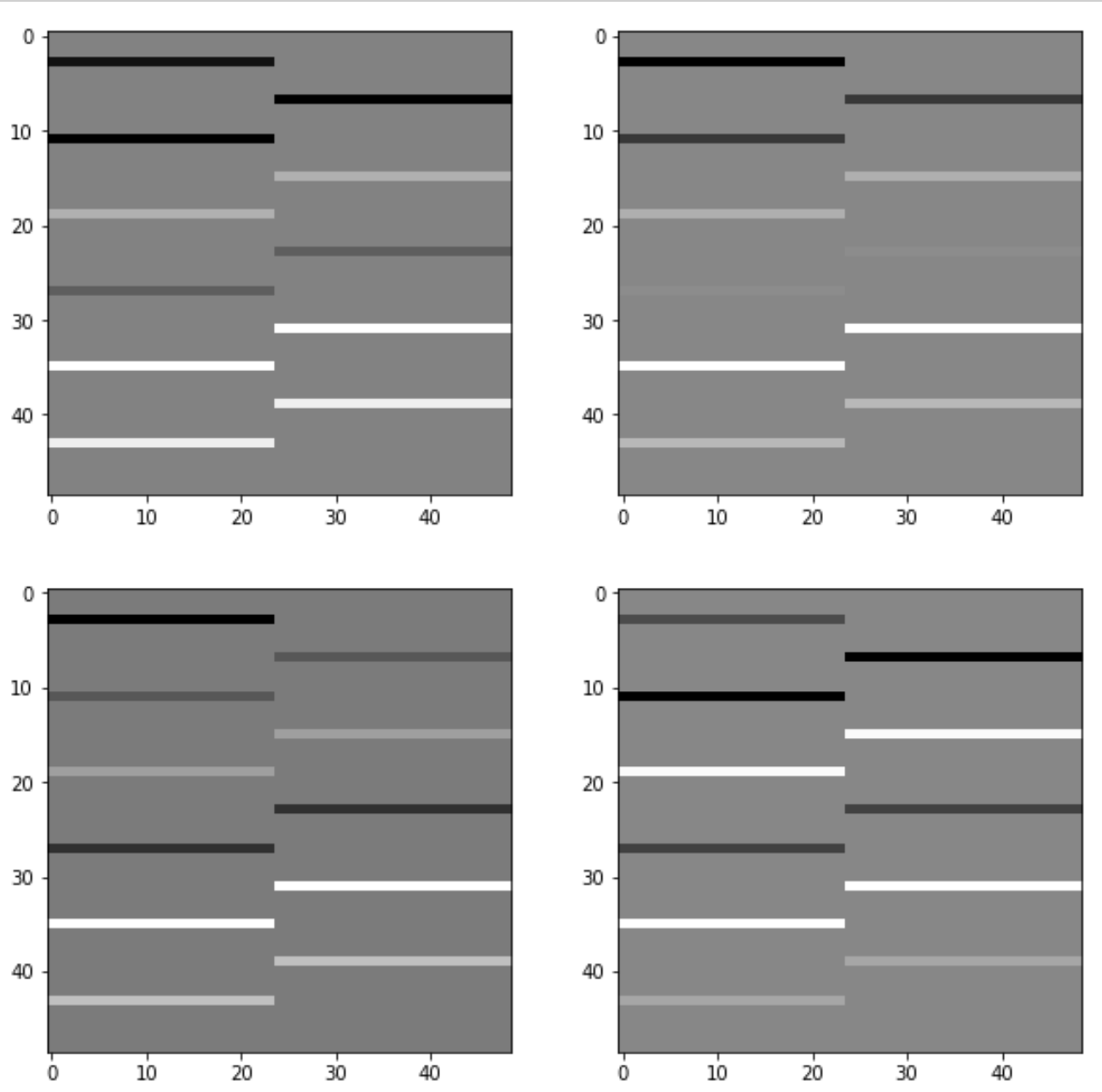Figure 4. Four reflection coefficients series.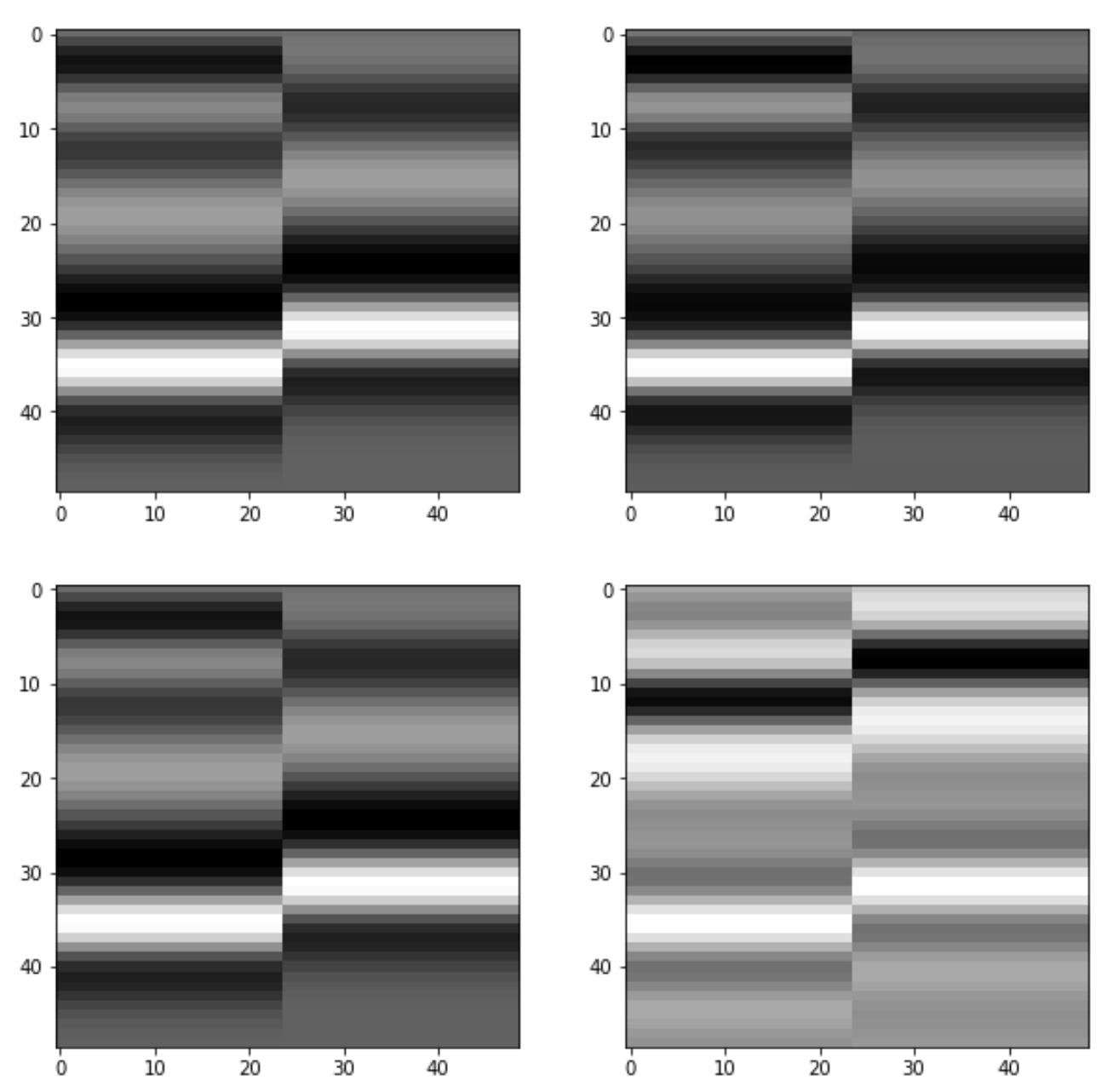Figure 5. Four synthetic seismic models with vertical fault.

The last bit is the addition of noise, with the result is shown in Figure 6:

```blurred = sp.ndimage.gaussian_filter(synth, sigma=1.1)
noisy = blurred + 0.5 * blurred.std() * np.random.random(blurred.shape)```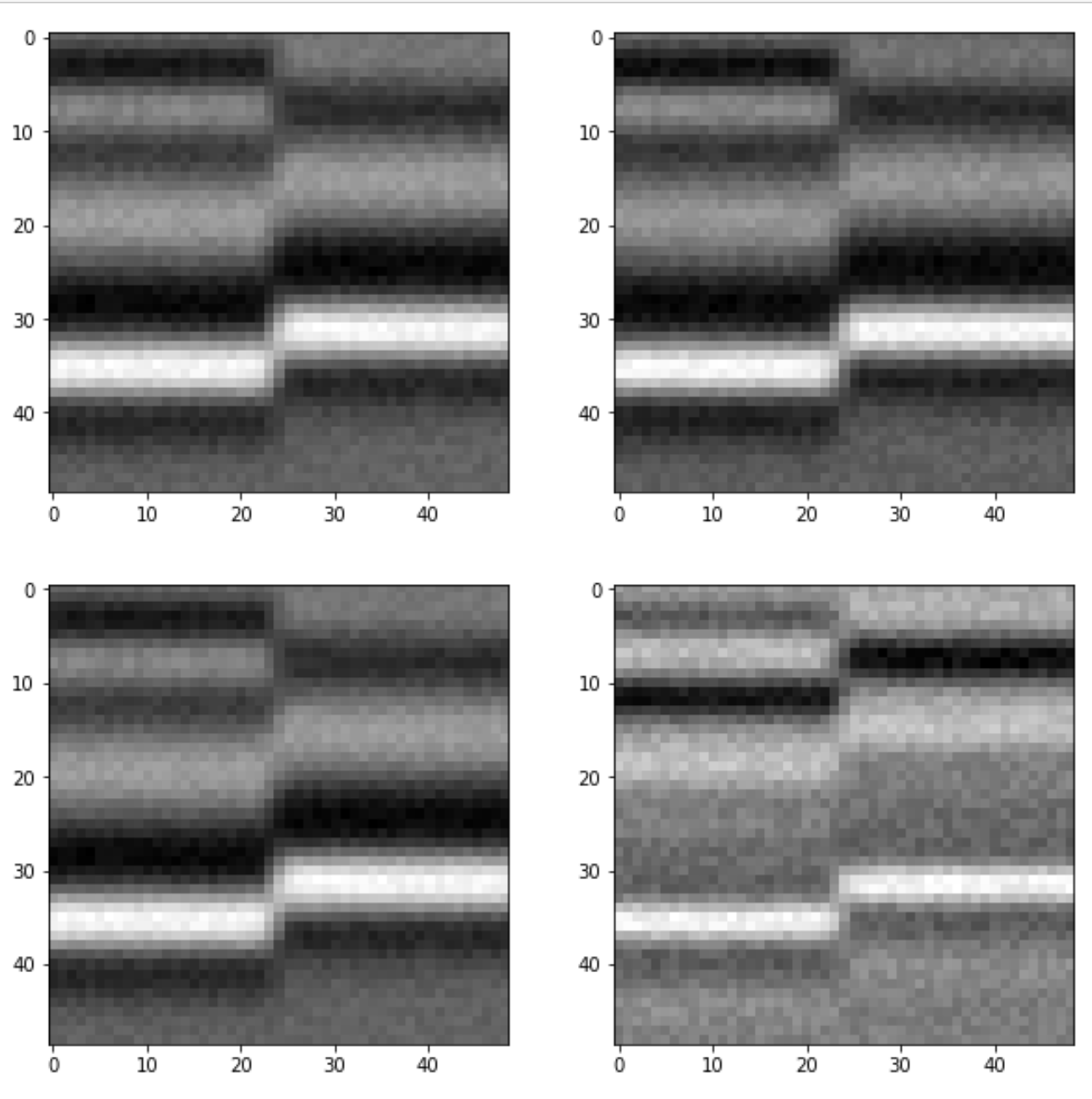Figure 6. Four synthetic seismic models with vertical fault and noise.

Done!

The notebook with the full code is on GitHub, let me know if you find this useful or if you come up with more modeling ideas.

UPDATE, April 22, 2019.

I think the key to understand this is how we multiply velocity by density in each of the possible earth model.

Looking at the notebook, the earth model array has shape:
```print (np.shape(earth))
>>> (10, 50, 49, 2)```
with the last axis having dimension 2: one Vp and one Rho, so in summary 10 models of size 50×49, each with a Vp and a Rho.
So with this other block of code:
```impedances = [np.apply_along_axis(np.product,
-1, e).astype(float) for e in earth]```

you use `numpy.apply_along_axis`  to multiply Vp by Rho along the last dimension, `-1` , using `numpy.product`, and the list comprehension `[... for e in earth]` to do it for all models in the array `earth`.